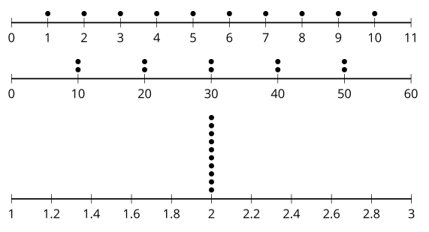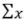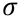# A.1.12.2 Investigating Standard Deviation (part 1)

Today you'll have the opportunity to investigate standard deviation. Standard deviation is another measure of variability (spread). It is calculted similar to the method used for MAD and we use standard deviation for variability when mean is the appropriate measure of center.
Enter the data for the first dot plot into the A column of the helper spreadsheet. Enter the data for the second dot plot into the B column of the helper spreadsheet. Enter the data for the third dot plot into the C column of the helper spreadsheet.Use the helper spreadsheet to find the Mean and the Standard Deviation for the data sets and list them: (to find standard deviation click the histogram icon, then click "One Variable Analysis" and finally click on theicon, the standard deviation symbol is this:)